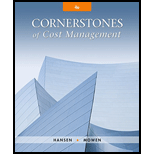# Refer to Cornerstone Exercise 9.1. Guillermo’s Oil and Lube Company provided the following information for the production of oil changes during the month of June: Actual number of oil changes performed: 980 Actual number of direct labor hours worked: 386 hours Actual rate paid per direct labor hour: $14.50 Standard rate per direct labor hour:$14.00 Required: 1. Calculate the direct labor rate variance ( LRV ) and the direct labor efficiency variance ( LEV ) for June using the formula approach. 2. Calculate the direct labor rate variance ( LRV ) and the direct labor efficiency variance ( LEV ) for June using the graphical approach. 3. Calculate the total direct labor variance for oil changes for June. 4. What if the actual wage rate paid in June was $12.40? What impact would that have had on the direct labor rate variance ( LRV )? On the direct labor efficiency variance ( LEV )?BuyFindarrow_forward ### Cornerstones of Cost Management (C... 4th Edition Don R. Hansen + 1 other Publisher: Cengage Learning ISBN: 9781305970663 #### Solutions Chapter SectionBuyFindarrow_forward ### Cornerstones of Cost Management (C... 4th Edition Don R. Hansen + 1 other Publisher: Cengage Learning ISBN: 9781305970663 Chapter 9, Problem 3CE Textbook Problem 142 views ## Refer to Cornerstone Exercise 9.1. Guillermo’s Oil and Lube Company provided the following information for the production of oil changes during the month of June:Actual number of oil changes performed: 980Actual number of direct labor hours worked: 386 hoursActual rate paid per direct labor hour:$14.50Standard rate per direct labor hour: $14.00Required: 1. Calculate the direct labor rate variance (LRV) and the direct labor efficiency variance (LEV) for June using the formula approach. 2. Calculate the direct labor rate variance (LRV) and the direct labor efficiency variance (LEV) for June using the graphical approach. 3. Calculate the total direct labor variance for oil changes for June. 4. What if the actual wage rate paid in June was$12.40? What impact would that have had on the direct labor rate variance (LRV)? On the direct labor efficiency variance (LEV)?

1.

To determine

Compute the direct labor rate variance and the direct labor efficiency variance for June (use formula approach).

### Explanation of Solution

Direct Labor Rate Variance

The direct labor rate variance is a measure to determine the variation in the estimated cost of the direct labor and the actual cost of the direct labor and is multiplied by the actual hours is called direct labor rate variance.

The following formula is used to calculate the direct labor rate variance:

Direct labor rate variance=[(ActualRateStandard Rate)×Actual Hours]

Compute the direct labor rate variance for June (use formula approach):

Direct labor rate variance=[(ActualRateStandard Rate)×Actual Hours]=[($14.50$14.00)×386Hours]=[$.50×386Hours]=$193 U

Direct labor efficiency variance is a measure that determines the difference between the estimated labor hours and the actual labor hours used and is multiplied by the standard rate per hour is called material usage variance

2.

To determine

Compute the direct labor rate variance and the direct labor efficiency variance for June (use graphical approach).

3.

To determine

Compute the total direct labor variance for June.

4.

To determine

Compute the direct labor rate variance and efficiency variance if the actual wage rate paid in June was \$12.40.

### Still sussing out bartleby?

Check out a sample textbook solution.

See a sample solution

#### The Solution to Your Study Problems

Bartleby provides explanations to thousands of textbook problems written by our experts, many with advanced degrees!

Get Started

Find more solutions based on key concepts
How are cost of goods sold and gross profit computed?

College Accounting, Chapters 1-27 (New in Accounting from Heintz and Parry)

Why do economists sometimes offer conflicting advice to policymakers?

Principles of Microeconomics (MindTap Course List)

In the Interim Financial Data section of the THOMSON FULL COMPANY RETORT, (scroll to the top and click on INTER...

Fundamentals of Financial Management, Concise Edition (with Thomson ONE - Business School Edition, 1 term (6 months) Printed Access Card) (MindTap Course List)

Explain the five types of discounts for business products.

Foundations of Business (MindTap Course List)

What is a VPN?

Accounting Information Systems